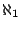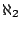# Representations of distributive algebraic lattices by lattices of two-sided ideals of rings, resp. submodule lattices of modules

Pavel Růžička

Charles University, Prague

We will present some results concerning representations of algebraic lattices in ideal lattices of rings, resp. submodule lattices of modules. We prove that any algebraic lattice which can be represented as the lattice of two-sided ideals of some ring can be represented as the submodule lattice of some module as well. Then we focus on algebraic distributive lattices. An algebraic distributive lattice is determined by the semilattice of its compact elements and these semilattices correspond to distributive-semilattices. We present the following results:

• Every distributive semilattices which is closed under finite meets is isomorphic to the semilattice of finitely generated two-sided ideals of some locally matricial algebra.
• Every countable distributive semilattices is isomorphic to the semilattice of finitely generated two-sided ideals of some locally matricial algebra.
• Every distributive semilattices of size at mostis isomorphic to the semilattice of finitely generated two-sided ideals of some von Neumann regular ring but there is a distributive semilattices of sizewhich is not isomorphic to the semilattice of two-sided ideals of any unit-regular ring, in particular, of any locally matricial algebra.
• There is a distributive semilattices of sizewhich is not isomorphic to the semilattice of finitely generated submodules of any module.

2005-05-23